• 针对Glitch PUF单元电路静态输出的缺陷，首次提出使用多层感知器(Multilayer Perceptron，MLP)算法对Glitch PUF进行机器学习，解决了Glitch PUF输出为非线性可分数据的问题，能够对Glitch PUF攻击并预测其输出。...信息安全
• 回归算法是统计机器学习的利器。常见的回归算法包括：最小二乘法（线性回归），逻辑回归，逐步式回归，多元自适应回归样条等。1.1 线性回归器所谓线性回归：对于一组输入值X=[x1,x2,…,xn]，存在输出y，为了代表输入...
【机器学习算法笔记】1. 回归器模型
回归算法是试图采用对误差的衡量来探索变量之间的关系的一类算法。回归算法是统计机器学习的利器。常见的回归算法包括：最小二乘法（线性回归），逻辑回归，逐步式回归，多元自适应回归样条等。
1.1 线性回归器
所谓线性回归：对于一组输入值X=[x1,x2,…,xn]，存在输出y，为了代表输入与输出的函数关系，假定输出估计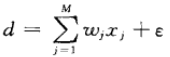线性回归器所要求的问题：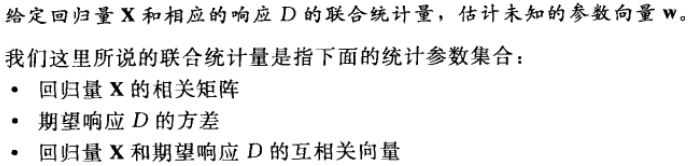一元线性回归的基本假设有  1、随机误差项是一个服从相同正态分布的彼此不相关随机变量，其期望值为0；  2、解释变量是确定性变量，不是随机变量，与随机误差项彼此之间相互独立；  3、解释变量之间不存在精确的（完全的）线性关系，即解释变量的样本观测值矩阵是满秩矩阵；  回归参数的最小二乘估计是一致最小方差无偏估计。
1.1.1 最大后验估计（MAP）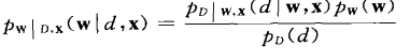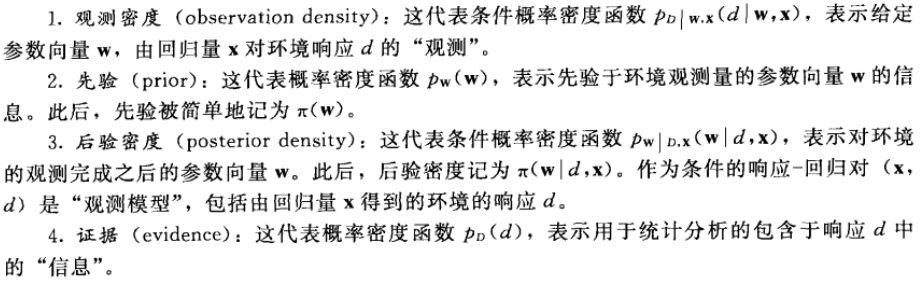上述建模所要达到的目的：给定参数向量w，使得观测概率最大！  所以我们需要建立观测密度的似然函数：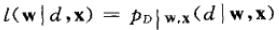然后求对W的最大似然估计（ML）：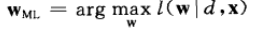而最大后验估计器（MAP）考虑到了W的后验概率，采用了参数向量所有的可能信息，并不忽略w的先验信息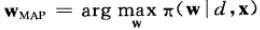高斯情况下的参数估计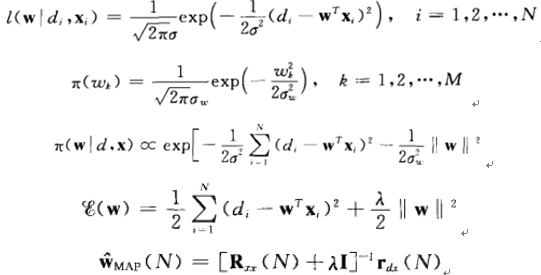通过利用正则化（即引入先验知识），来改进最大似然估计器的稳定性，其最大后验估计器是有偏的。
1.1.2 最小二乘估计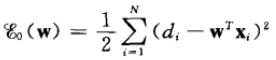可以看出最小二乘估计同高斯估计下的ML是相似的，回归参数的最小二乘估计是一致最小方差无偏估计，同理，为了得到唯一性和稳定性的解，添加了正则项：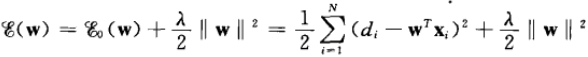正则最小二乘估计同MAP是相似的。
1.2 感知器
感知器是用于线性可分模式分类的最简单的神经网络模型。  Rosenblatt感知器如下图所示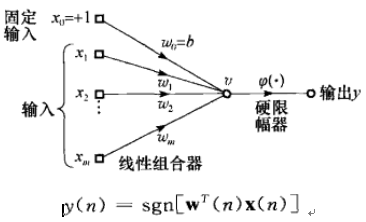权值向量的自适应更新：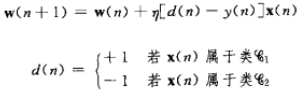1.3 逻辑回归模型(Logistic Regression, LR)
1.3.1逻辑回归含义
Logistic回归与多重线性回归实际上有很多相同之处，最大的区别就在于它们的因变量不同，其他的基本都差不多。正是因为如此，这两种回归可以归于同一个家族，即广义线性模型。这一家族中的模型形式基本上都差不多，不同的就是因变量不同：
如果是连续的，就是多重线性回归；如果是二项分布，就是Logistic回归；如果是Poisson分布，就是Poisson回归；如果是负二项分布，就是负二项回归。
Logistic回归主要针对于解决因变量是二分类的问题。Logistic回归的主要用途：
寻找危险因素：寻找某一疾病的危险因素等；预测：根据模型，预测在不同的自变量情况下，发生某病或某种情况的概率有多大；判别：实际上跟预测有些类似，也是根据模型，判断某人属于某病或属于某种情况的概率有多大，也就是看一下这个人有多大的可能性是属于某病。这里的因变量就是是否胃癌，即“是”或“否”，自变量就可以包括很多了，例如年龄、性别、饮食习惯、幽门螺杆菌感染等。自变量既可以是连续的，也可以是分类的。
1.3.2 逻辑回归模型
逻辑回归的模型是一个非线性模型，但是它本质上又是一个线性回归模型，又或者说是感知器模型。其相当于在普通线性模型输出后，再增加了一个sigmoid函数，又称逻辑回归函数。又或者相当于在感知器模型的sign函数用sigmoid函数来代替。  Logistic regression是针对于二分类问题的，所学习的系统的方程为，这里的h指的是分类正确概率：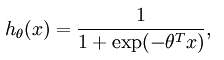其对应的损失函数为：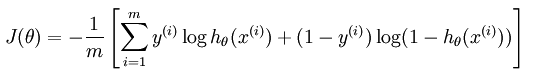添加正则项后的目标函数：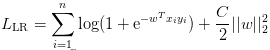1.3.2 逻辑回归解释
从最小熵上考虑  函数h(θ)的值有特殊的含义，它表示结果取1的概率，因此对于输入x分类结果为类别1和类别0的概率分别为：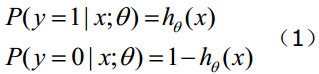从最大似然角度上考虑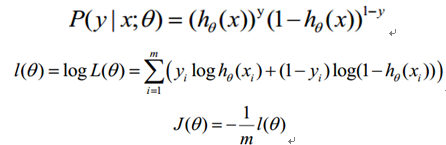求解方法  仍然是梯度下降法：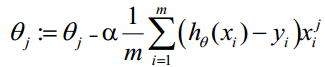1.4 Softmax回归模型
上节可以看出逻辑回归是针对于二分类问题的，如果现在的假设是多分类问题，比如说总共有k个类别。这个时候就需要softmax回归，其是逻辑回归在多分类上的推广。  在softmax regression中这时候的系统的方程为：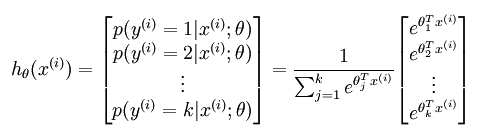其中的参数θ不再是列向量，而是一个矩阵，矩阵的每一行可以看做是一个类别所对应分类器的参数，总共有k行。所以矩阵θ可以写成下面的形式：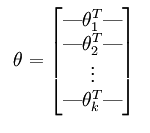此时，系统损失函数的方程为：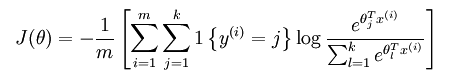其中的1{.}是一个指示性函数，即当大括号中的值为真时，该函数的结果就为1，否则其结果就为0。softmax regression中损失函数的偏导函数如下所示：公式表示的是损失函数对第j个类别的参数的偏导。所以上面的公式还只是一个类别的偏导公式，我们需要求出所有类别的偏导公式。  实际上，softmax回归中对参数的最优化求解通常不只一个，每当求得一个优化参数时，如果将这个参数的每一项都减掉同一个数，其得到的损失函数值也是一样的。这说明这个参数不是唯一解。用数学公式证明过程如下所示：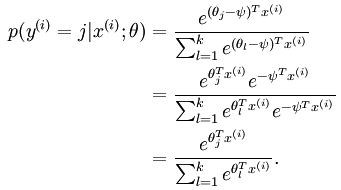那这个到底是什么原因呢？从宏观上可以这么理解，因为此时的损失函数不是严格非凸的，也就是说在局部最小值点附近是一个“平坦”的，所以在这个参数附近的值都是一样的了。那么怎样避免这个问题呢？其实加入正则项就可以解决（比如说，用牛顿法求解时，hession矩阵如果没有加入规则项，就有可能不是可逆的从而导致了刚才的情况，如果加入了规则项后该hession矩阵就不会不可逆了），加入规则项后的损失函数表达式如下：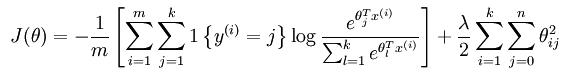这个时候的偏导函数表达式如下所示：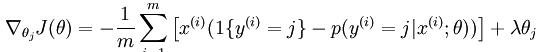接下来剩下的问题就是用数学优化的方法来求解了。
1.5 HK算法
HK算法思想很朴实,就是在最小均方误差准则下求得权矢量。  他相对于感知器算法的优点在于，他适用于线性可分和非线性可分得情况，对于线性可分的情况，给出最优权矢量，对于非线性可分得情况，能够判别出来，以退出迭代过程。
1.5.1 关于收敛条件的判决：
对于误差矢量：e=x*w-b  若e>0 则继续迭代  若e=0 则停止迭代，得到权矢量  若e<0 则停止迭代，样本是非线性可分得，  若e有的分量大于0，有的分量小于0 ，则在各分量都变成零，或者停止由负值转变成正值时，停机。
1.6 MLP多层感知器
多层感知器的特征：  1、网络中每个神经元包含了一个可微的非线性激活函数  2、网络中包含了一个或多个隐藏在输入和输出神经节点之间的层  3、网络表现出高度的连接性，其强度是由网络的突触权重所决定  要点：  反向传播法  批量学习：多个样例构成一个回合，代价函数是由平均误差能量来定义的，能够精确估计梯度向量。  在线学习：代价函数是由全体瞬时误差能量，容易执行，不容易陷入局部极值点，存储空间少，速度要慢。  机器学习中常见误差：  1、逼近误差：这是给定训练样本的固定大小N时，由训练神经网络或者机器学习所招致的误差  2、估计误差：用以前没有出现过的数据测试其性能所招致的误差  3、最优化误差：在给定计算时间下，训练机器的计算精确度所引起的

这篇博文是个人的学习笔记，内容许多来源于网络（包括CSDN、博客园及百度百科等），博主主要做了些微不足道的整理工作。由于在做笔记的时候没有注明来源，所以如果有作者看到上述文字中有自己的原创内容，请私信本人修改或注明来源，非常感谢>_<

展开全文• 正则化、MLE、MLP三个串联起来 阐述线性回归从两个方面： 未加正则化的线性回归： ①标量的最小二乘法LSE： 损失函数是 L=∣∣wTxi−yi∣∣2 L = ||w^Tx_i-y_i||^2 L=∣∣wTxi​−yi​∣∣2 ，目标是求其最小值，对...


关于线性回归正则化、MLE、MLP
正则化、MLE、MLP阐述线性回归从两个方面：未加正则化的线性回归：加了正则化后的线性回归：过拟合：正则化的框架：另：加L2正则化（矩阵形式）加L2正则化（MLE-概率形式-频率派）MLE如下：加L2正则化（概率形式-贝叶斯派）

结论

正则化、MLE、MLP
阐述线性回归从两个方面：
未加正则化的线性回归：
①标量的最小二乘法LSE：
损失函数是

L

=

∣

∣

w

T

x

i

−

y

i

∣

∣

2

L = ||w^Tx_i-y_i||^2

，目标是求其最小值，对其做矩阵变换，变换后对w求导，

∂

L

(

w

)

w

=

(

X

T

X

+

X

T

X

)

W

−

X

T

Y

−

X

T

Y

=

2

X

T

X

W

−

2

X

T

Y

=

0

\begin{aligned} \frac{\partial L(w)}{w} &=(X^TX+X^TX)W-X^TY-X^TY \\ &=2X^TXW-2X^TY=0 \end{aligned}

得参数w的解，
有解即有最小值，但由于解的形式带逆，因此结果有风险
②概率角度解释：
MLE，假设值+高斯，概率
加了正则化后的线性回归：
最小二乘法 《=》最大后验估计
即 MLE+正则化 = MLP

过拟合：
①增加数据
②减少模型复杂度：特征选取pca、方差、P值
③正则化
正则化的框架：

a

r

g

m

i

n

[

L

(

w

)

+

λ

P

(

w

)

]

argmin[L(w)+\lambda P(w)]

，其中

L

(

w

)

L(w)

是损失函数，

P

(

w

)

P(w)

是惩罚项

另：

P

(

w

)

=

∣

∣

w

1

∣

∣

1

P(w)=||w_1||_1

L

1

约

束

范

围

−

>

∣

w

1

∣

+

∣

w

1

∣

+

.

.

.

+

∣

w

n

∣

<

=

C

L1约束范围->|w_1|+|w_1|+...+|w_n|<=CP

(

w

)

=

∣

∣

w

2

∣

∣

1

2

=

w

T

w

P(w)=||w_2||^2_1=w^Tw

L

2

约

束

范

围

−

>

∣

w

1

∣

2

+

∣

w

1

∣

2

+

.

.

.

+

∣

w

n

∣

2

<

=

C

L2约束范围->\sqrt{|w_1|^2+|w_1|^2+...+|w_n|^2}<=C加L2正则化（矩阵形式）

因

为

P

(

w

)

=

w

T

w

所

以

P

(

w

)

′

=

2

λ

w

\begin{aligned} 因为P(w)=w^Tw \\ 所以P(w)'=2\lambda w \end{aligned}

其中w是n维的向量，加了L2正则化的求导如下

∂

L

(

w

)

w

=

(

X

T

X

+

X

T

X

)

W

−

X

T

Y

−

X

T

Y

+

2

λ

w

=

2

(

X

T

X

+

λ

I

)

W

−

X

T

Y

解

得

w

=

(

X

T

X

+

λ

I

)

−

1

X

T

Y

\begin{aligned} \frac{\partial L(w)}{w} &=(X^TX+X^TX)W-X^TY-X^TY+2\lambda w \\ &=2(X^TX+\lambda I)W-X^TY \end{aligned} \\ 解得w = (X^TX+\lambda I)^{-1}X^TY

加L2正则化（MLE-概率形式-频率派）
MLE如下：
已知

y

=

w

T

x

+

ε

，

ε

∼

N

(

0

,

σ

2

)

y=w^Tx+\varepsilon，\varepsilon \sim N(0,\sigma^2)

得

p

=

(

y

∣

x

i

;

w

)

∼

N

(

w

T

x

,

σ

2

)

p=(y|x_i;w)\sim N(w^Tx,\sigma^2)

而对于单个样本来说

p

(

y

∣

w

)

=

1

2

π

σ

e

−

1

2

σ

2

(

y

i

−

w

T

x

i

)

2

p(y|w) = \frac{1}{\sqrt{2 \pi} \sigma} e^{-\frac{1}{2 \sigma^{2}}(y_i-w^Tx_i)^{2}}

又MLE是为了求得某参数使得函数达到最大值，这里我们用连乘
MLE->max(p1 * p2 * p3…pn)
因此，对于N个样本来说，概率似然函数为：

L

(

w

)

=

l

o

g

P

(

Y

∣

X

;

w

)

=

l

o

g

∏

i

=

1

N

P

(

y

i

∣

x

i

;

w

)

=

∏

i

=

1

N

l

o

g

P

(

y

i

∣

x

i

;

w

)

=

Σ

i

=

1

N

l

o

g

1

2

π

σ

+

Σ

i

=

1

N

l

o

g

e

−

1

2

σ

2

(

y

i

−

w

T

x

i

)

2

=

Σ

i

=

1

N

(

l

o

g

1

2

π

σ

−

1

2

σ

2

(

y

i

−

w

T

x

i

)

2

)

\begin{aligned} L(w)&=log{P(Y|X;w)} \\&=log\prod_{i=1}^{N}{P(y_i|x_i;w)} \\&=\prod_{i=1}^{N}log{P(y_i|x_i;w)} \\&=\Sigma_{i=1}^{N}log\frac{1}{\sqrt{2 \pi} \sigma} + \Sigma_{i=1}^{N}loge^{-\frac{1}{2 \sigma^{2}}(y_i-w^Tx_i)^{2}} \\&=\Sigma_{i=1}^{N}(log\frac{1}{\sqrt{2 \pi} \sigma}-\frac{1}{2 \sigma^{2}}(y_i-w^Tx_i)^2) \end{aligned}

求解参数w为

w

^

=

a

r

g

m

a

x

(

L

(

w

)

)

=

a

r

g

m

a

x

(

−

1

2

σ

2

(

y

i

−

w

T

x

i

)

2

)

=

a

r

g

m

i

n

(

y

i

−

w

T

x

i

)

2

\begin{aligned}\hat{w}&=argmax(L(w)) \\&=argmax(-\frac{1}{2 \sigma^{2}}(y_i-w^Tx_i)^2) \\&=argmin(y_i-w^Tx_i)^2 \end{aligned}

得出：最小二乘法隐含了一个噪声服从正态分布的假设
加L2正则化（概率形式-贝叶斯派）
此时的w参数不再是固定的，而是一个随机变量，根据新增信息(先验)

w

∼

N

(

0

,

σ

0

2

)

w \sim N(0,\sigma_0^2)

计算修正概率，更新后验概率值得到后验概率

p

(

w

∣

y

i

)

=

p

(

y

∣

w

)

p

(

w

)

p

(

y

)

p(w|y_i)=\frac{p(y|w)p(w)}{p(y)}

MAP角度来看，求w的最大后验:

w

^

=

a

r

g

m

a

x

(

∏

i

=

1

N

p

(

w

∣

y

i

)

)

\begin{aligned}\hat{w}&=argmax(\prod_{i=1}^{N}p(w|y_i)) \end{aligned}

因为p(y)跟w无关，相当于一个常量，因此有

w

^

=

a

r

g

m

a

x

(

p

(

y

∣

w

i

)

p

(

w

i

)

)

\begin{aligned} \\\hat{w}&=argmax(p(y|w_i)p(w_i)) \end{aligned}

又由前面MLE可得（单样本来看）

似

然

：

p

(

y

∣

w

)

=

1

2

π

σ

2

e

−

1

2

σ

2

(

y

i

−

w

T

x

i

)

2

似然：p(y|w) = \frac{1}{\sqrt{2 \pi} \sigma^2} e^{-\frac{1}{2 \sigma^{2}}(y_i-w^Tx_i)^{2}}

先

验

：

p

(

w

)

=

1

2

π

σ

0

2

e

−

1

2

σ

2

∣

∣

w

∣

∣

2

2

先验：p(w) = \frac{1}{\sqrt{2 \pi} \sigma_0^2} e^{-\frac{1}{2 \sigma^{2}}||w||_2^{2}}

可得

w

^

=

a

r

g

m

a

x

(

p

(

y

∣

w

)

p

(

w

)

)

=

a

r

g

m

a

x

l

o

g

[

p

(

y

∣

w

)

p

(

w

)

]

=

a

r

g

m

a

x

l

o

g

(

p

(

y

∣

w

)

)

+

a

r

g

m

a

x

l

o

g

(

p

(

w

)

)

=

a

r

g

m

a

x

(

−

1

2

σ

2

(

y

−

w

T

x

)

2

−

1

2

σ

0

2

∣

∣

w

∣

∣

2

2

)

=

a

r

g

m

i

n

(

(

y

−

w

T

x

)

2

σ

2

+

∣

∣

w

∣

∣

2

2

σ

0

2

)

\begin{aligned} \\\hat{w}&=argmax(p(y|w)p(w)) \\&=argmaxlog[p(y|w)p(w)] \\&=argmaxlog(p(y|w))+argmaxlog(p(w)) \\&=argmax(-\frac{1}{2 \sigma^{2}}(y-w^Tx)^{2}-\frac{1}{2 \sigma_0^{2}}||w||_2^{2}) \\&=argmin(\frac{(y-w^Tx)^{2}}{\sigma^{2}}+\frac{||w||_2^{2}}{\sigma_0^{2}}) \end{aligned}

因此

w

^

M

A

P

=

a

r

g

m

i

n

Σ

i

=

1

N

(

y

i

−

w

T

x

i

)

2

+

σ

2

σ

0

2

∣

∣

w

∣

∣

2

2

\begin{aligned} \\\hat{w}_{MAP}&=argmin\Sigma_{i=1}^{N}(y_i-w^Tx_i)^{2}+\frac{\sigma^{2}}{\sigma_0^{2}}||w||_2^{2} \end{aligned}

结论
结论1：未加正则项情况下，最小二乘法是 《=》极大似然估计MLE+噪声符合高斯分布  结论2：加L2正则项情况下，最小二乘法/MLE是 《=》MAP+先验和噪声都符合高斯分布 
展开全文• 这里使用的机器学习算法包括：人工神经网络，boost,决策树，最近邻，逻辑回归，贝叶斯，随机森林，SVM等算法等。机器学习的过程相同，都要经历1、收集样本数据sampleData2.训练分类器mode3.对测试数据testData进行...
opencv提供了非常多的机器学习算法用于研究。这里对这些算法进行分类学习和研究，以抛砖引玉。这里使用的机器学习算法包括：人工神经网络，boost,决策树，最近邻，逻辑回归，贝叶斯，随机森林，SVM等算法等。
机器学习的过程相同，都要经历1、收集样本数据sampleData2.训练分类器mode3.对测试数据testData进行预测
这里使用一个在别处看到的例子，利用身高体重等原始信息预测男女的概率。通过一些简单的数据学习，用测试数据预测男女概率。

import org.opencv.core.Core;
import org.opencv.core.CvType;
import org.opencv.core.Mat;
import org.opencv.core.TermCriteria;
import org.opencv.ml.ANN_MLP;
import org.opencv.ml.Boost;
import org.opencv.ml.DTrees;
import org.opencv.ml.KNearest;
import org.opencv.ml.LogisticRegression;
import org.opencv.ml.Ml;
import org.opencv.ml.NormalBayesClassifier;
import org.opencv.ml.RTrees;
import org.opencv.ml.SVM;
import org.opencv.ml.SVMSGD;
import org.opencv.ml.TrainData;

public class ML {
public static void main(String[] args) {
// 训练数据，两个维度，表示身高和体重
float[] trainingData = { 186, 80, 185, 81, 160, 50, 161, 48 };
// 训练标签数据，前两个表示男生0，后两个表示女生1,由于使用了多种机器学习算法，他们的输入有些不一样，所以labelsMat有三种
float[] labels = { 0f, 0f, 0f, 0f, 1f, 1f, 1f, 1f };
int[] labels2 = { 0, 0, 1, 1 };
float[] labels3 = { 0, 0, 1, 1 };
// 测试数据,先男后女
float[] test = { 184, 79, 159, 50 };

Mat trainingDataMat = new Mat(4, 2, CvType.CV_32FC1);
trainingDataMat.put(0, 0, trainingData);

Mat labelsMat = new Mat(4, 2, CvType.CV_32FC1);
labelsMat.put(0, 0, labels);

Mat labelsMat2 = new Mat(4, 1, CvType.CV_32SC1);
labelsMat2.put(0, 0, labels2);

Mat labelsMat3 = new Mat(4, 1, CvType.CV_32FC1);
labelsMat3.put(0, 0, labels3);

Mat sampleMat = new Mat(2, 2, CvType.CV_32FC1);
sampleMat.put(0, 0, test);

MyAnn(trainingDataMat, labelsMat, sampleMat);
MyBoost(trainingDataMat, labelsMat2, sampleMat);
MyDtrees(trainingDataMat, labelsMat2, sampleMat);
MyKnn(trainingDataMat, labelsMat3, sampleMat);
MyLogisticRegression(trainingDataMat, labelsMat3, sampleMat);
MyNormalBayes(trainingDataMat, labelsMat2, sampleMat);
MyRTrees(trainingDataMat, labelsMat2, sampleMat);
MySvm(trainingDataMat, labelsMat2, sampleMat);
MySvmsgd(trainingDataMat, labelsMat2, sampleMat);
}

// 人工神经网络
public static Mat MyAnn(Mat trainingData, Mat labels, Mat testData) {
// train data using aNN
TrainData td = TrainData.create(trainingData, Ml.ROW_SAMPLE, labels);
Mat layerSizes = new Mat(1, 4, CvType.CV_32FC1);
// 含有两个隐含层的网络结构，输入、输出层各两个节点，每个隐含层含两个节点
layerSizes.put(0, 0, new float[] { 2, 2, 2, 2 });
ANN_MLP ann = ANN_MLP.create();
ann.setLayerSizes(layerSizes);
ann.setTrainMethod(ANN_MLP.BACKPROP);
ann.setBackpropWeightScale(0.1);
ann.setBackpropMomentumScale(0.1);
ann.setActivationFunction(ANN_MLP.SIGMOID_SYM, 1, 1);
ann.setTermCriteria(new TermCriteria(TermCriteria.MAX_ITER + TermCriteria.EPS, 300, 0.0));
boolean success = ann.train(td.getSamples(), Ml.ROW_SAMPLE, td.getResponses());
System.out.println("Ann training result: " + success);
// ann.save("D:/bp.xml");//存储模型

// 测试数据
Mat responseMat = new Mat();
ann.predict(testData, responseMat, 0);
System.out.println("Ann responseMat:\n" + responseMat.dump());
for (int i = 0; i < responseMat.size().height; i++) {
if (responseMat.get(i, 0) + responseMat.get(i, i) >= 1)
System.out.println("Girl\n");
if (responseMat.get(i, 0) + responseMat.get(i, i) < 1)
System.out.println("Boy\n");
}
return responseMat;
}

// Boost
public static Mat MyBoost(Mat trainingData, Mat labels, Mat testData) {
Boost boost = Boost.create();
// boost.setBoostType(Boost.DISCRETE);
boost.setBoostType(Boost.GENTLE);
boost.setWeakCount(2);
boost.setWeightTrimRate(0.95);
boost.setMaxDepth(2);
boost.setUseSurrogates(false);
boost.setPriors(new Mat());

TrainData td = TrainData.create(trainingData, Ml.ROW_SAMPLE, labels);
boolean success = boost.train(td.getSamples(), Ml.ROW_SAMPLE, td.getResponses());
System.out.println("Boost training result: " + success);
// boost.save("D:/bp.xml");//存储模型

Mat responseMat = new Mat();
float response = boost.predict(testData, responseMat, 0);
System.out.println("Boost responseMat:\n" + responseMat.dump());
for (int i = 0; i < responseMat.height(); i++) {
if (responseMat.get(i, 0) == 0)
System.out.println("Boy\n");
if (responseMat.get(i, 0) == 1)
System.out.println("Girl\n");
}
return responseMat;
}

// 决策树
public static Mat MyDtrees(Mat trainingData, Mat labels, Mat testData) {
DTrees dtree = DTrees.create(); // 创建分类器
dtree.setMaxDepth(8); // 设置最大深度
dtree.setMinSampleCount(2);
dtree.setUseSurrogates(false);
dtree.setCVFolds(0); // 交叉验证
dtree.setUse1SERule(false);
dtree.setTruncatePrunedTree(false);

TrainData td = TrainData.create(trainingData, Ml.ROW_SAMPLE, labels);
boolean success = dtree.train(td.getSamples(), Ml.ROW_SAMPLE, td.getResponses());
System.out.println("Dtrees training result: " + success);
// dtree.save("D:/bp.xml");//存储模型

Mat responseMat = new Mat();
float response = dtree.predict(testData, responseMat, 0);
System.out.println("Dtrees responseMat:\n" + responseMat.dump());
for (int i = 0; i < responseMat.height(); i++) {
if (responseMat.get(i, 0) == 0)
System.out.println("Boy\n");
if (responseMat.get(i, 0) == 1)
System.out.println("Girl\n");
}
return responseMat;
}

// K最邻近
public static Mat MyKnn(Mat trainingData, Mat labels, Mat testData) {
final int K = 2;
TrainData td = TrainData.create(trainingData, Ml.ROW_SAMPLE, labels);
KNearest knn = KNearest.create();
boolean success = knn.train(trainingData, Ml.ROW_SAMPLE, labels);
System.out.println("Knn training result: " + success);
// knn.save("D:/bp.xml");//存储模型

// find the nearest neighbours of test data
Mat results = new Mat();
Mat neighborResponses = new Mat();
Mat dists = new Mat();
knn.findNearest(testData, K, results, neighborResponses, dists);
System.out.println("results:\n" + results.dump());
System.out.println("Knn neighborResponses:\n" + neighborResponses.dump());
System.out.println("dists:\n" + dists.dump());
for (int i = 0; i < results.height(); i++) {
if (results.get(i, 0) == 0)
System.out.println("Boy\n");
if (results.get(i, 0) == 1)
System.out.println("Girl\n");
}

return results;
}

// 逻辑回归
public static Mat MyLogisticRegression(Mat trainingData, Mat labels, Mat testData) {
LogisticRegression lr = LogisticRegression.create();

TrainData td = TrainData.create(trainingData, Ml.ROW_SAMPLE, labels);
boolean success = lr.train(td.getSamples(), Ml.ROW_SAMPLE, td.getResponses());
System.out.println("LogisticRegression training result: " + success);
// lr.save("D:/bp.xml");//存储模型

Mat responseMat = new Mat();
float response = lr.predict(testData, responseMat, 0);
System.out.println("LogisticRegression responseMat:\n" + responseMat.dump());
for (int i = 0; i < responseMat.height(); i++) {
if (responseMat.get(i, 0) == 0)
System.out.println("Boy\n");
if (responseMat.get(i, 0) == 1)
System.out.println("Girl\n");
}
return responseMat;
}

// 贝叶斯
public static Mat MyNormalBayes(Mat trainingData, Mat labels, Mat testData) {
NormalBayesClassifier nb = NormalBayesClassifier.create();

TrainData td = TrainData.create(trainingData, Ml.ROW_SAMPLE, labels);
boolean success = nb.train(td.getSamples(), Ml.ROW_SAMPLE, td.getResponses());
System.out.println("NormalBayes training result: " + success);
// nb.save("D:/bp.xml");//存储模型

Mat responseMat = new Mat();
float response = nb.predict(testData, responseMat, 0);
System.out.println("NormalBayes responseMat:\n" + responseMat.dump());
for (int i = 0; i < responseMat.height(); i++) {
if (responseMat.get(i, 0) == 0)
System.out.println("Boy\n");
if (responseMat.get(i, 0) == 1)
System.out.println("Girl\n");
}
return responseMat;
}

// 随机森林
public static Mat MyRTrees(Mat trainingData, Mat labels, Mat testData) {
RTrees rtrees = RTrees.create();
rtrees.setMaxDepth(4);
rtrees.setMinSampleCount(2);
rtrees.setRegressionAccuracy(0.f);
rtrees.setUseSurrogates(false);
rtrees.setMaxCategories(16);
rtrees.setPriors(new Mat());
rtrees.setCalculateVarImportance(false);
rtrees.setActiveVarCount(1);
rtrees.setTermCriteria(new TermCriteria(TermCriteria.MAX_ITER, 5, 0));
TrainData tData = TrainData.create(trainingData, Ml.ROW_SAMPLE, labels);
boolean success = rtrees.train(tData.getSamples(), Ml.ROW_SAMPLE, tData.getResponses());
System.out.println("Rtrees training result: " + success);
// rtrees.save("D:/bp.xml");//存储模型

Mat responseMat = new Mat();
rtrees.predict(testData, responseMat, 0);
System.out.println("Rtrees responseMat:\n" + responseMat.dump());
for (int i = 0; i < responseMat.height(); i++) {
if (responseMat.get(i, 0) == 0)
System.out.println("Boy\n");
if (responseMat.get(i, 0) == 1)
System.out.println("Girl\n");
}
return responseMat;
}

// 支持向量机
public static Mat MySvm(Mat trainingData, Mat labels, Mat testData) {
SVM svm = SVM.create();
svm.setKernel(SVM.LINEAR);
svm.setType(SVM.C_SVC);
TermCriteria criteria = new TermCriteria(TermCriteria.EPS + TermCriteria.MAX_ITER, 1000, 0);
svm.setTermCriteria(criteria);
svm.setGamma(0.5);
svm.setNu(0.5);
svm.setC(1);

TrainData td = TrainData.create(trainingData, Ml.ROW_SAMPLE, labels);
boolean success = svm.train(td.getSamples(), Ml.ROW_SAMPLE, td.getResponses());
System.out.println("Svm training result: " + success);
// svm.save("D:/bp.xml");//存储模型

Mat responseMat = new Mat();
svm.predict(testData, responseMat, 0);
System.out.println("SVM responseMat:\n" + responseMat.dump());
for (int i = 0; i < responseMat.height(); i++) {
if (responseMat.get(i, 0) == 0)
System.out.println("Boy\n");
if (responseMat.get(i, 0) == 1)
System.out.println("Girl\n");
}
return responseMat;
}

// SGD支持向量机
public static Mat MySvmsgd(Mat trainingData, Mat labels, Mat testData) {
SVMSGD Svmsgd = SVMSGD.create();
TermCriteria criteria = new TermCriteria(TermCriteria.EPS + TermCriteria.MAX_ITER, 1000, 0);
Svmsgd.setTermCriteria(criteria);
Svmsgd.setInitialStepSize(2);
Svmsgd.setSvmsgdType(SVMSGD.SGD);
Svmsgd.setMarginRegularization(0.5f);
boolean success = Svmsgd.train(trainingData, Ml.ROW_SAMPLE, labels);
System.out.println("SVMSGD training result: " + success);
// svm.save("D:/bp.xml");//存储模型

Mat responseMat = new Mat();
Svmsgd.predict(testData, responseMat, 0);
System.out.println("SVMSGD responseMat:\n" + responseMat.dump());
for (int i = 0; i < responseMat.height(); i++) {
if (responseMat.get(i, 0) == 0)
System.out.println("Boy\n");
if (responseMat.get(i, 0) == 1)
System.out.println("Girl\n");
}
return responseMat;
}
}

输出结果：

Ann training result: true
Ann responseMat:
[0.014712702, 0.01492399;
0.98786205, 0.987822]
Boy

Girl

Boost training result: true
Boost responseMat:
[0;
0]
Boy

Boy

Dtrees training result: true
Dtrees responseMat:
[0;
1]
Boy

Girl

Knn training result: true
results:
[0;
1]
Knn neighborResponses:
[0, 0;
1, 1]
dists:
[5, 5;
1, 8]
Boy

Girl

LogisticRegression training result: true
LogisticRegression responseMat:
[0;
1]
Boy

Girl

NormalBayes training result: true
NormalBayes responseMat:
[0;
1]
Boy

Girl

Rtrees training result: true
Rtrees responseMat:
[0;
1]
Boy

Girl

Svm training result: true
SVM responseMat:
[0;
1]
Boy

Girl

SVMSGD training result: true
SVMSGD responseMat:
[1;
1]
Girl

Girl



参考：
http://www.cnblogs.com/denny402/p/5032232.html
http://www.cnblogs.com/denny402/p/5032490.html

展开全文opencv java boost
• 机器学习的一项作业，仅供参考，主要KNN、逻辑回归、SVM、mlp、cnn、朴素贝叶斯的分类器性能的比较
• 机器学习分类以及一些常见算法的简单说明
机器学习分类
监督学习：传统意义上的机器学习，即训练数据有标签，机器在学习过程中是有反馈的，知道正确与否；无监督学习：与监督学习相反，没有标签，也没有反馈，只能从数据本身去挖掘，寻找规律；强化学习：通过与环境进行交互获得的奖赏指导行为，目标是使智能体获得最大的奖赏；半监督学习：顾名思义，介于监督学习和无监督学习之间；深度学习：通过多层神经网络提取特征，提取特征数量大种类多，再根据反馈去调整网络参数。
python的机器学习库sklearn
1.自带数据集：2.sklearn的主要功能：
功能算法聚类K均值聚类K-means、DBSCAN、高斯混合模型GMM、BRICH、谱聚类、AP聚类、均值漂移、层次聚类降维主成分分析PCA、独立成分分析FastICA、非负矩阵分解NMF、LDA、字典学习、因子分析分类k邻近kNN、支持向量机SVM、朴素贝叶斯naivebayes、决策树、集成方法、MLP回归普通线性回归、岭回归、Lasso回归、弹性网络、最小角回归、贝叶斯回归、逻辑回归、多项式回归模型选择略数据预处理略
常用算法
1.无监督学习
无监督学习一般分为聚类和降维
（1）聚类（clustering）：对无标签的一组数据进行聚类，通过其“相似度”，也就是“距离”进行分类，通常分类的类别数是可以调整的。
距离包括
欧氏距离（传统的平面几何距离，可拓展到高维空间）曼哈顿距离（街区距离）马氏距离（协方差距离）夹角余弦距离（向量的相似度）
算法
K-means：随机找k个点作为初始聚类中心；对于剩下的点，以此计算其余点与聚类中心的距离（上面提到的，通常为高维空间），根据其与聚类中心的距离，归入最近的簇；对每个簇，计算所有点的均值作为新的聚类中心；重复以上步骤，直到聚类中心不发生改变。DBSCAN：基于密度的算法，不需要人为指定簇的个数，故最终簇的个数不确定。将数据点分为3类： ——核心点：在给定半径内含有超过确定个数的点，即临近点比较多 ——边界点：在核心点的邻域内，但不满足核心点的要求 ——噪音点：不满足以上条件 流程为将所有点标记为核心点、边界点或者噪音点；删除噪音点；在给定距离内的核心点赋予边；每组连通的核心点形成一个簇；将边界点分配到与其关联的核心点的簇中。例子：比如目前有全国30个城市的几个方面的数据，例如在衣、食、住、行4个方面的消费，要把30个城市分成几个不同的类别，就要用到聚类算法。
（2）降维（decomposition）：尽可能保证原本数据特征不变的情况下，将高维数据转化为低维数据，一般用作数据的可视化，或者减轻后续计算的数据量。
算法
PCA：将线性相关的高维变量合成线性无关的低维向量，称为主成分，主成分尽可能保留原始数据的信息。简单来说，矩阵的主成分就是其协方差矩阵对应的特征向量，按照大小，找出前m个（就是我们要降到的低维个数）特征值。NMF：非负矩阵分解，原理就是非负矩阵可以分解为两个非负矩阵的乘积。例子：鸢尾花是一个常见的数据集，但是其是4个指标，即4维，无法直接看出其空间分布，于是需要降维将其转化为2维平面上的点，易于观察。
2.监督学习
监督学习即利用有标签的数据，学习其规律，目的是对未知数据进行很好的预测，一般分为分类和回归。监督学习都会有训练集和测试集之分。
（1）分类：输出离散即为分类。根据对训练集的学习，面对测试集可以准确将其分到所属类别，有二分类，也有多分类。
算法
kNN：通过计算一个数据点与所有数据点之间的距离，根据给定的邻居个数N，取出其邻居各自的类别，它的邻居哪一类最多，就判断此样本点属于哪一类。 k的取值很关键，较大时虽然会根据更多的样本点来判断，但是也会因为引入距离较远的样本，从而导致预测错误；较小时，容易出现过拟合现象，容易被噪音点影响。决策树：西瓜书的第一个模型。通常问题会被诸多因素影响，而我们的目的是产生一个最合适的判断模型，只要顺着树枝就可以得到正确的结果。朴素贝叶斯：首先概率论中学过贝叶斯定理，朴素贝叶斯就是为了求出后验概率最大的y，作为其分类结果。假设某一类的样本点服从一个Gaussian Distribution（已知均值和协方差可以得到概率分布的函数），然后求出这2个参数，之后对于测试集的数据，只需要计算其后验概率即可。例如，二分类问题中，大于0.5和小于0.5视为不同的2类。MLP：即多层感知机。最简单的2层神经网络也叫做感知机，中间加入隐含层后即为多层感知机，是一种很简单的神经网络。例子：根据对数据集中的猫和狗的学习，从而产生合适的分类器，对未知图片可以判断其是猫还是狗。
（2）回归：输出连续即为回归。与分类类似，只不过是输出为连续的情况，根据训练集去预测，对于输入可以给出一个合适的数值。自变量（特征）只有一个时为一元回归，多个时为多元回归。
算法
简单回归多项式回归岭回归：上述二者都是基于最小二乘法进行拟合，岭回归相比较而言是一种改良的最小二乘法。
例子：最简单的回归即为散点图的回归，下图给了一个二维的例子，高维可以类比；回归也可以分为线性和非线性。个人看来回归更倾向于预测，例如股票（当然这个很难预测）、交通流量预测，可以理解为就是对时间序列进行拟合，只不过将序列替换为高维的概念。
3.强化学习
强化学习是一种试错学习，通过环境提供的反馈调整策略，最终达到奖赏最大的目的。
算法
马尔可夫决策过程MDP：和信息论中的马尔可夫链很像，根据当前状态和转移概率求得下一个状态，是基于模型的算法。蒙特卡洛强化学习：当环境中状态太多时，学习算法不再依赖于环境建模，即为免模型算法。这里提到了贪心策略。Q-learning：结合动态规划和蒙特卡洛强化学习。深度强化学习DRL：将深度学习和传统强化学习结合，也避免了状态过多的问题，直接从环境（输入）和动作（输出）去学习。DQN：Deep Q Network，DL+Q-learning
推荐资料
书籍： 《机器学习》-周志华: 主页. 《PRML》-Bishop: 主页.
课程： 《Python机器学习应用》-中国大学MOOC： 课程主页. 《Machine Learning》-Andrew Ng： Coursera版课程主页. Stanford手书版在线观看.（这个网页404了） Stanford手书版课程主页. 《CS231n》-Fei Fei Li： 在线观看.（这个网页也404了） 课程主页. 《Reinforcement Learning》-David Silver： 课程主页. 在线观看.
展开全文• 希望通过所给的训练数据学习一个贷款申请的决策树，用以对未来的贷款申请进行分类，即当新的客户提出贷款申请时，根据申请人的特征利用决策树决定是否批准贷款申请。 在编写代码之前，我们先对数据集进行属性...
• 机器学习算法总结1 分类回归算法1.1 逻辑回归（LR）1.2 Softmax回归1.3 因子分解机（FM）1.4 支持向量机（SVM）1.5 决策树1.6 K 最近邻算法（KNN）1.7 岭回归和Lasso回归2 聚类算法2.1 K-Means2.2 均值漂移算法...
• 今天开始总结和复习机器学习知识点： 一句话概括： 1. 聚类：无监督学习，学习结果将产生几个集合，集合中的元素彼此相似； 2. 分类：有监督学习，学习结果将产生几个函数，通过函数划分为几个集合，数据对象是...
• 本系列文章参考了《机器学习Python实践》，会通过例子一步一步地引导大家使用机器学习来处理和分类与回归模型相关的问题。每个算法模型都介绍其较为通用且实用的建模过程，力争使基础较差的读者也能无障碍利用python...python
• 参考链接：...KNN 回归` knn回归 from sklearn import neighbors knn_reg = neighbors.KNeighborsRegressor(40, weights=‘uniform’, metric=‘euclidean’) %time knn_reg.fit(o...KNN SVM
• 机器学习(Machine Learning, ML)是一门多领域交叉学科，涉及概率论、统计学、逼近论、凸分析、算法复杂度理论等多门学科。专门研究计算机怎样模拟或实现人类的学习行为，以获取新的知识或技能，重新组织已有的知识...
• 在之前的【【深度学习】DNN神经网络模型】一文中弄清楚MLP基础由来，本次进一步基于sklearn机器学习库来实现该过程。神经网络 多层感知机 全连接神经网络
• 机器学习算法分为： 有监督，无监督 两大阵营 1.有监督： 单变量线性回归，多变量线性回归，逻辑回归，多项式回归，神经网络，支持向量机，决策树，KNN，朴素贝叶斯，集成学习，等 2.无监督： 聚类：K-Means,K-Means...集成学习 笔记
• 很多人在平时的工作中都或多或少会用到机器学习算法。本文为您总结一下常见的机器学习算法，以供您在工作和学习中参考。  机器学习算法很多。很多时候困惑人们都是，很多算法是一类算法，而有些算法又是...
• ## BAT机器学习面试1000题系列

万次阅读 多人点赞 2017-12-14 15:19:15
1、本文的内容全部来源于七月在线发布的BAT机器学习面试1000题系列； 2、文章中带斜体的文字代表是本人自己增加的内容，如有错误还请批评指正； 3、原文中有部分链接已经失效，故而本人重新加上了新的链接，如有...深度学习 程序员
• 可以用于回归和分类问题的多层感知机(MLP)，是深度学习的一种算法，被称为（普通）前馈神经网络。 神经网络模型 MLP被视为广义的线性模型，多次重复计算加权求和的过程，并将激活函数用于加权求和，得到输出。 激活...神经网络
• 集成算法详解前言一、随机森林算法原理二、随机森林的优势与特征重要性指标1.随机森林的优势2.特征重要性指标三、提升算法概述四、堆叠...前一篇博客对决策树算法进行了详解，属于建立模型的基础，如果想要机器学习决策树 python 集成学习
• 机器学习常用算法——分类器效果展示 注：仅个人成果展示，效果不可直接用于判断算法好坏 原图 1、决策树 2、K-means 3、KNN 4、LDA 5、逻辑回归 6、随机森林 7、SVM 8、MLP
• 多层感知器（MLP） Rosenblatt感知器和LMS算法，都是单层的并且是单个神经元构造的神经网络，他们的局限性是只能解决线性可分问题，例如Rosenblatt感知器一直没办法处理简单异或问题。然而日常生活中大多数问题...多层感知器
• 在目前的机器学习领域中，最常见的三种任务就是：回归分析、分类分析、聚类分析。那么什么是回归呢？ 回归分析是一种预测性的建模技术，它研究的...常用回归算法 这里介绍一些回归问题中常用的机器学习方法，sklea...
• 本系列文章参考了《机器学习Python实践》，会通过例子一步一步地引导大家使用机器学习来处理和分类与回归模型相关的问题。每个算法模型都介绍其较为通用且实用的建模过程，力争使基础较差的读者也能无障碍利用python...
• 【导语】：本文推荐的 GitHub 项目用 Python 实现流行的机器学习算法，还包括算法实现背后的解析。每个算法都有交互式 Jupyter Notebook 演示，你可以使用它来训练...python 人工智能 深度学习
• 回归器)组合之后产生一个新学习器。 弱分类器(weak learner)指那些分类准确率只稍微好于随机猜测的分类器(error rate < 0.5)； 集成算法的成功在于保证弱分类器的多样性(Diversity)。而且集成不稳定的算法 也能够...python
•  Boosting, 也称为增强学习或提升法，是一种重要的集成学习技术， 能够将预测精度仅比随机猜度略高的弱学习器增强为预测精度高的强学习器，这在直接构造强学习器非常困难的情况下，为学习算法的设计提供了一种有效...AdaBoost...